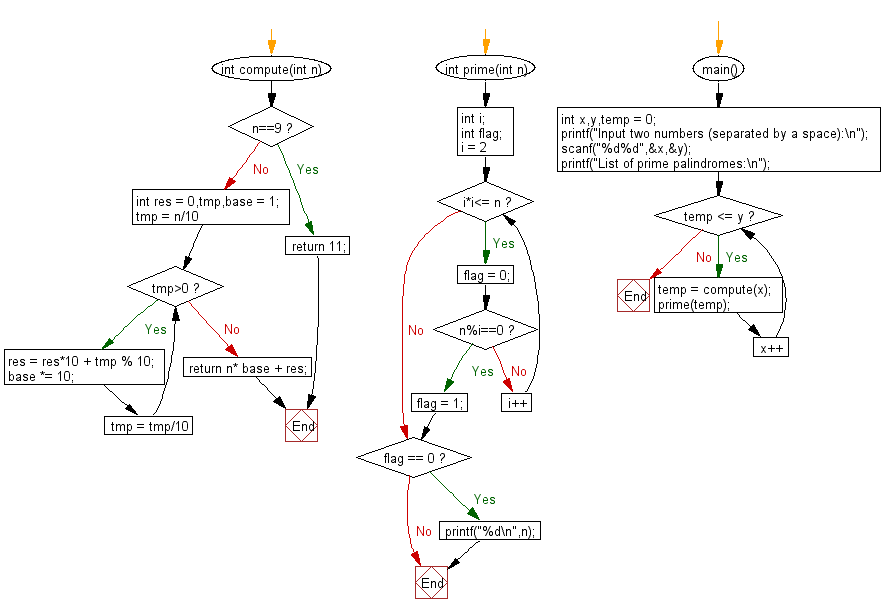﻿ C Program: Find all prime palindromes between x and y

# C Exercises: Find all prime palindromes in the range of two given numbers x and y

## C Basic Declarations and Expressions: Exercise-90 with Solution

Write a C program to find all prime palindromes in the range of two given numbers x and y (5 <= x<y<= 1000,000,000).
A number is called a prime palindrome if the number is both a prime number and a palindrome.

Sample Solution:

C Code:

``````#include<stdio.h>

// Function to compute a palindrome number from a given number
int compute(int n){
if(n == 9) // Special case for n = 9
return 11; // Return 11
int res = 0, tmp, base = 1;

// Loop to reverse the digits of n
for(tmp = n / 10; tmp> 0; tmp = tmp / 10){
res = res * 10 + tmp % 10; // Build the reversed number
base *= 10;
}

return n * base + res; // Combine the original and reversed numbers
}

// Function to check if a number is prime and print it if it is
int prime(int n){
int i;
int flag;

// Loop to check for factors of n
for(i = 2; i * i<= n; i++){
flag = 0;
if(n % i == 0){
flag = 1; // Set flag to indicate non-prime
break;
}
}

if(flag == 0){ // If flag remains 0, n is a prime number
printf("%d\n", n); // Print the prime number
}
}

int main(){
int x, y, temp = 0;

printf("Input two numbers (separated by a space):\n");
scanf("%d %d", &x, &y);

printf("List of prime palindromes:\n");

// Loop to generate and check palindrome numbers
for(; temp <= y; x++){
temp = compute(x);
prime(temp);
}

return 0; // Indicate successful execution of the program
}
``````

Sample Output:

```Input two numbers (separated by a space):
List of prime palindromes:
0
1
```

Flowchart:C programming Code Editor:

What is the difficulty level of this exercise?

Test your Programming skills with w3resource's quiz.

﻿